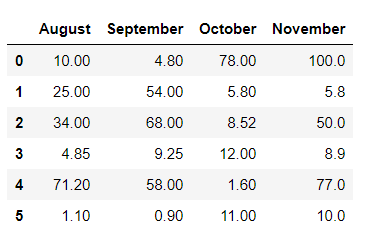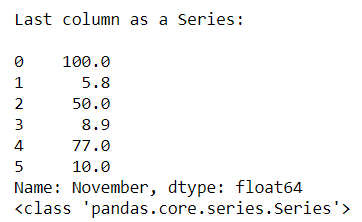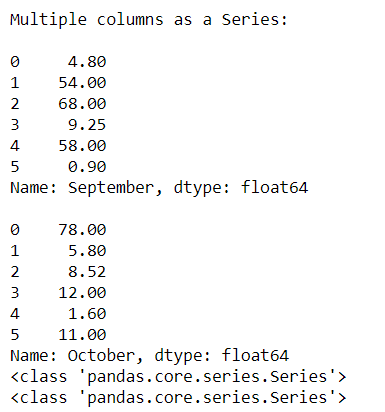# How to Convert Pandas DataFrame columns to a Series?

It is possible in pandas to convert columns of the pandas Data frame to series. Sometimes there is a need to converting columns of the data frame to another type like series for analyzing the data set.

Case 1: Converting the first column of the data frame to Series

## Python3

 `# Importing pandas module ` `import` `pandas as pd ` ` `  `# Creating a dictionary  ` `dit ``=` `{``'August'``: [``10``, ``25``, ``34``, ``4.85``, ``71.2``, ``1.1``],  ` `       ``'September'``: [``4.8``, ``54``, ``68``, ``9.25``, ``58``, ``0.9``],  ` `       ``'October'``: [``78``, ``5.8``, ``8.52``, ``12``, ``1.6``, ``11``],  ` `       ``'November'``: [``100``, ``5.8``, ``50``, ``8.9``, ``77``, ``10``] } ` ` `  `# Converting it to data frame ` `df ``=` `pd.DataFrame(data``=``dit) ` ` `  `# Original DataFrame ` `df`

Output:Converting the first column to series.

## Python3

 `# Converting first column i.e 'August' to Series ` `ser1 ``=` `df.ix[:,``0``] ` ` `  `print``(``"\n1st column as a Series:\n"``) ` `print``(ser1) ` ` `  `# Checking type ` `print``(``type``(ser1))`

Output:In the above example, we change the type of column ‘August‘ from the data frame to Series.

Case 2: Converting the last column of the data frame to Series

## Python3

 `# Importing pandas module ` `import` `pandas as pd ` ` `  `# Creating a dictionary  ` `dit ``=` `{``'August'``: [``10``, ``25``, ``34``, ``4.85``, ``71.2``, ``1.1``],  ` `       ``'September'``: [``4.8``, ``54``, ``68``, ``9.25``, ``58``, ``0.9``],  ` `       ``'October'``: [``78``, ``5.8``, ``8.52``, ``12``, ``1.6``, ``11``],  ` `       ``'November'``: [``100``, ``5.8``, ``50``, ``8.9``, ``77``, ``10``] } ` ` `  `# Converting it to data frame ` `df ``=` `pd.DataFrame(data``=``dit) ` ` `  `# Original DataFrame ` `df `

Output:Converting the last column to the series.

## Python3

 `# Converting last column i.e 'November' to Series ` `ser1 ``=` `df.ix[:,``3``] ` ` `  `print``(``"\nLast column as a Series:\n"``) ` `print``(ser1) ` ` `  `# Checking type ` `print``(``type``(ser1)) `

Output:In the above example, we change the type of column ‘November‘ from the data frame to Series.

Case 3: Converting the multiple columns of the data frame to Series

## Python3

 `# Importing pandas module ` `import` `pandas as pd ` ` `  `# Creating a dictionary  ` `dit ``=` `{``'August'``: [``10``, ``25``, ``34``, ``4.85``, ``71.2``, ``1.1``],  ` `       ``'September'``: [``4.8``, ``54``, ``68``, ``9.25``, ``58``, ``0.9``],  ` `       ``'October'``: [``78``, ``5.8``, ``8.52``, ``12``, ``1.6``, ``11``],  ` `       ``'November'``: [``100``, ``5.8``, ``50``, ``8.9``, ``77``, ``10``] } ` ` `  `# Converting it to data frame ` `df ``=` `pd.DataFrame(data``=``dit) ` ` `  `# Original DataFrame ` `df `

Output:Converting multiple columns to series.

## Python3

 `# Converting multiple columns  ` `# i.e 'September' and 'October' to Series ` `ser1 ``=` `df.ix[:,``1``] ` `ser2 ``=` `df.ix[:,``2``] ` ` `  `print``(``"\nMultiple columns as a Series:\n"``) ` `print``(ser1) ` `print``() ` `print``(ser2) ` ` `  `# Checking type ` `print``(``type``(ser1)) ` `print``(``type``(ser2))`

Output:In the above example, we change the type of 2 columns i.e ‘September‘ and ‘October’ from the data frame to Series.

Whether you're preparing for your first job interview or aiming to upskill in this ever-evolving tech landscape, GeeksforGeeks Courses are your key to success. We provide top-quality content at affordable prices, all geared towards accelerating your growth in a time-bound manner. Join the millions we've already empowered, and we're here to do the same for you. Don't miss out - check it out now!

Previous
Next